# Ziggy Baby Changing Pad Cover, Cradle Bassinet Sheets Fitted Jersey Cotton , Blue/Grey,

Ziggy Baby
•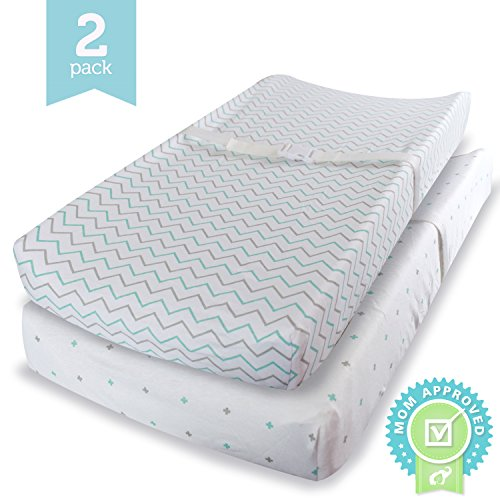•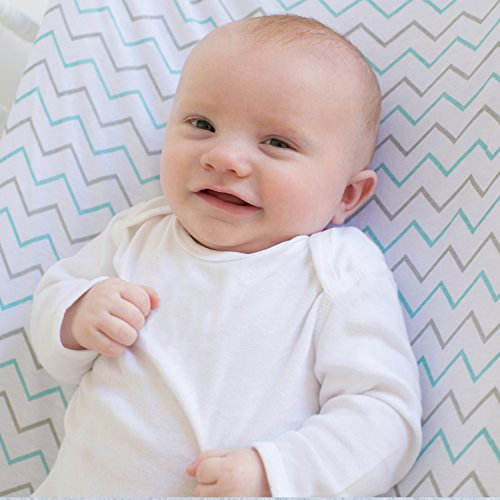•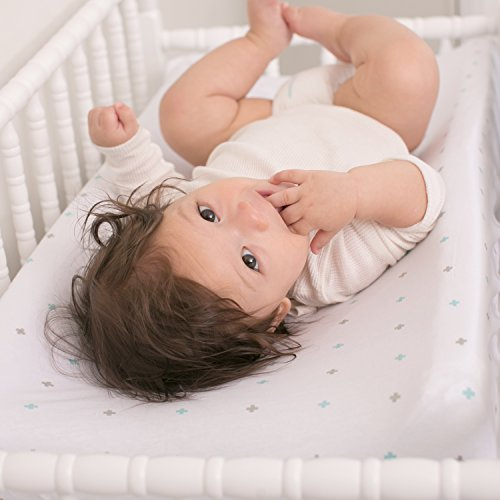•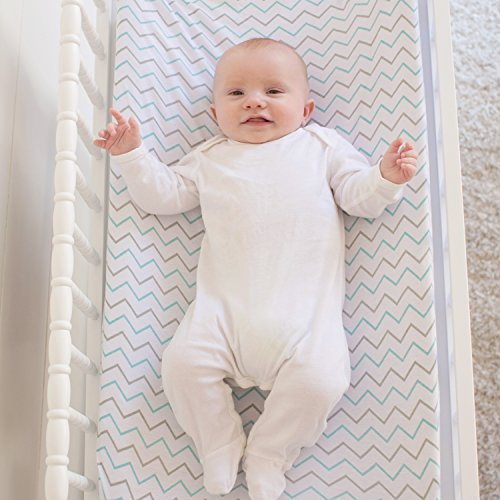•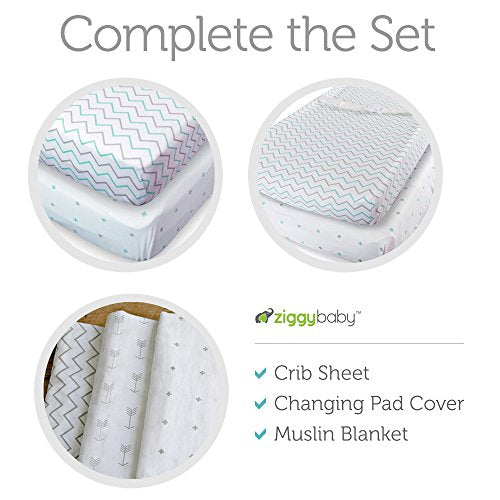•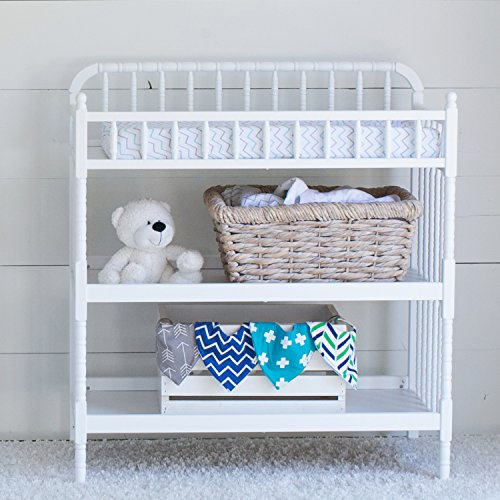•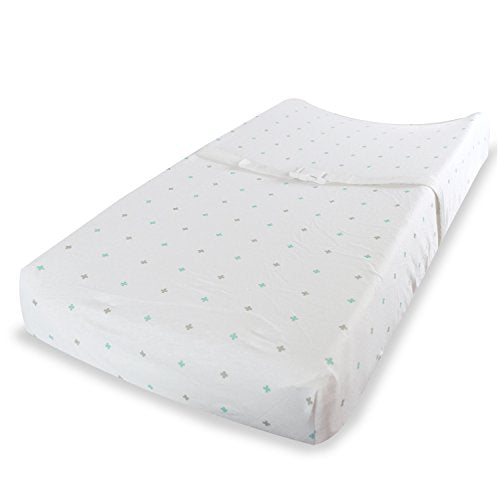•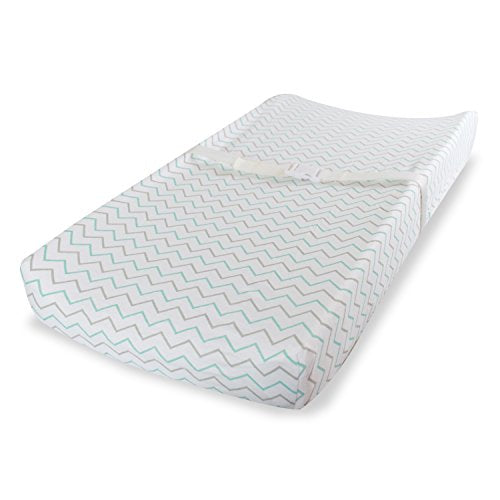\$33.99 \$45.18
25% OFF.### Free shipping

Free shipping on all orders.### Free Returns

Free 30 Days Returns. Returns & Refund Policy### Secure Shopping Guarantee

We use Secure Sockets Layer (SSL) technology to provide you with the safest, most secure shopping experience possible.

QTY:
• U

• N

• I

• V

• E

• R

• S

• A

• L

• F

• I

• T

• ,

• F

• U

• L

• L

• Y

• E

• L

• A

• S

• T

• I

• C

• I

• Z

• E

• D

• Z

• i

• g

• g

• y

• B

• a

• b

• y

• '

• s

• 3

• 2

• x

• 1

• 6

• x

• 5

• i

• n

• c

• h

• n

• u

• r

• s

• e

• r

• y

• b

• e

• d

• d

• i

• n

• g

• s

• e

• t

• s

• s

• e

• c

• u

• r

• e

• l

• y

• f

• i

• t

• m

• o

• s

• t

• s

• t

• a

• n

• d

• a

• r

• d

• c

• o

• n

• t

• o

• u

• r

• e

• d

• c

• h

• a

• n

• g

• i

• n

• g

• p

• a

• d

• s

• a

• n

• d

• f

• l

• a

• t

• c

• h

• a

• n

• g

• i

• n

• g

• t

• a

• b

• l

• e

• p

• a

• d

• s

• ,

• b

• a

• s

• s

• i

• n

• e

• t

• m

• a

• t

• t

• r

• e

• s

• s

• ,

• a

• n

• d

• c

• r

• a

• d

• l

• e

• m

• a

• t

• t

• r

• e

• s

• s

• ^

• 1

• 0

• 0

• %

• J

• E

• R

• S

• E

• Y

• K

• N

• I

• T

• C

• O

• T

• T

• O

• N

• O

• u

• r

• s

• u

• p

• e

• r

• s

• o

• f

• t

• b

• a

• b

• y

• s

• h

• e

• e

• t

• s

• c

• o

• n

• t

• a

• i

• n

• a

• 2

• p

• a

• c

• k

• o

• f

• p

• r

• e

• m

• i

• u

• m

• c

• o

• t

• t

• o

• n

• f

• i

• t

• t

• e

• d

• s

• h

• e

• e

• t

• s

• f

• r

• o

• m

• o

• u

• r

• B

• l

• u

• e

• C

• o

• l

• l

• e

• c

• t

• i

• o

• n

• .

• C

• o

• m

• p

• l

• e

• t

• e

• t

• h

• e

• s

• e

• t

• w

• i

• t

• h

• C

• r

• i

• b

• S

• h

• e

• e

• t

• s

• a

• n

• d

• M

• u

• s

• l

• i

• n

• S

• w

• a

• d

• d

• l

• e

• B

• l

• a

• n

• k

• e

• t

• s

• ^

• F

• A

• S

• H

• I

• O

• N

• A

• B

• L

• E

• ,

• F

• U

• N

• C

• T

• I

• O

• N

• A

• L

• O

• u

• r

• b

• a

• b

• y

• b

• e

• d

• d

• i

• n

• g

• s

• e

• t

• s

• f

• o

• r

• b

• o

• y

• s

• o

• r

• g

• i

• r

• l

• s

• a

• r

• e

• t

• r

• e

• n

• d

• y

• y

• e

• t

• f

• u

• n

• c

• t

• i

• o

• n

• a

• l

• a

• s

• c

• h

• a

• n

• g

• i

• n

• g

• p

• a

• d

• s

• h

• e

• e

• t

• s

• ,

• b

• a

• s

• s

• i

• n

• e

• t

• s

• h

• e

• e

• t

• s

• ,

• a

• n

• d

• c

• r

• a

• d

• l

• e

• s

• h

• e

• e

• t

• s

• f

• o

• r

• y

• o

• u

• r

• b

• a

• b

• y

• n

• u

• r

• s

• e

• r

• y

• o

• r

• a

• s

• b

• a

• b

• y

• s

• h

• o

• w

• e

• r

• g

• i

• f

• t

• s

• e

• t

• s

• ^

• M

• A

• C

• H

• I

• N

• E

• W

• A

• S

• H

• A

• B

• L

• E

• S

• i

• m

• p

• l

• y

• t

• o

• s

• s

• o

• u

• r

• d

• u

• r

• a

• b

• l

• e

• b

• a

• b

• y

• n

• u

• r

• s

• e

• r

• y

• s

• h

• e

• e

• t

• s

• i

• n

• t

• h

• e

• w

• a

• s

• h

• t

• o

• c

• l

• e

• a

• n

• .

• O

• u

• r

• 2

• p

• a

• c

• k

• g

• i

• v

• e

• s

• y

• o

• u

• a

• n

• e

• x

• t

• r

• a

• c

• o

• v

• e

• r

• ,

• s

• o

• y

• o

• u

• '

• l

• l

• a

• l

• w

• a

• y

• s

• h

• a

• v

• e

• a

• s

• p

• a

• r

• e

• f

• o

• r

• y

• o

• u

• r

• b

• a

• b

• y

• d

• i

• a

• p

• e

• r

• c

• h

• a

• n

• g

• i

• n

• g

• s

• t

• a

• t

• i

• o

• n

• ^

• Z

• I

• G

• G

• Y

• B

• A

• B

• Y

• P

• R

• O

• M

• I

• S

• E

• W

• i

• t

• h

• o

• v

• e

• r

• 2

• ,

• 3

• 0

• 0

• s

• e

• l

• l

• e

• r

• r

• e

• v

• i

• e

• w

• s

• ,

• w

• e

• h

• o

• l

• d

• a

• 4

• .

• 9

• s

• t

• a

• r

• r

• a

• t

• i

• n

• g

• b

• e

• c

• a

• u

• s

• e

• w

• e

• c

• a

• r

• e

• a

• b

• o

• u

• t

• c

• u

• s

• t

• o

• m

• e

• r

• s

• e

• r

• v

• i

• c

• e

• .

• W

• e

• h

• o

• n

• o

• r

• a

• N

• o

• Q

• u

• e

• s

• t

• i

• o

• n

• s

• A

• s

• k

• e

• d

• R

• e

• t

• u

• r

• n

• P

• o

• l

• i

• c

• y

• s

• o

• y

• o

• u

• c

• a

• n

• A

• d

• d

• T

• o

• C

• a

• r

• t

• W

• i

• t

• h

• C

• o

• n

• f

• i

• d

• e

• n

• c

• e

• Color : Blue/Grey